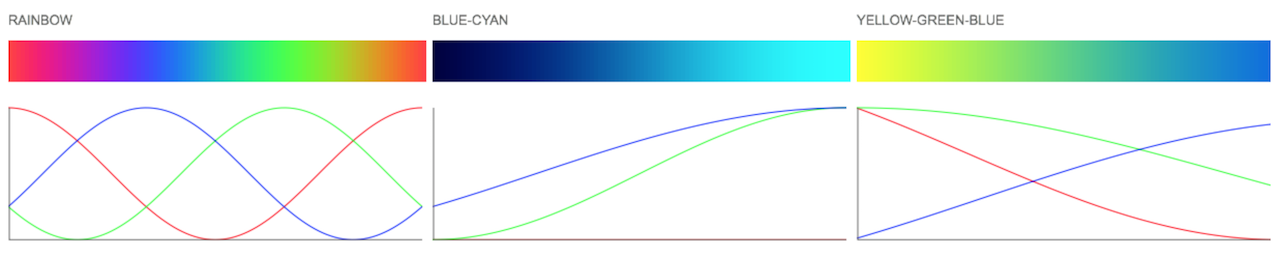## UsageParameters:
`scheme` - array of cosine coefficients for R,G,B channels

Returns:
`function(t)` - a function calculating a color value for t=0..1. The returned color is an array `[0..1, 0..1, 0..1]`

## Example

To get the middle blue gradient from the screenshot you would:

``````var cosineGradient = require('cosine-gradient');
var scheme = [
[0.000,0.500,0.500],
[0.000,0.500,0.500],
[0.000,0.500,0.333],
[0.000,0.500,0.667]
];

for(var i=0; i<=100; i++) {
var t = i / 100;
var color = gradient(t); //[R=0..1, G=0..1, B=0..1]
}
``````

## Credits

This is an implementation of a technique by Inigo Quilez (Color Palettes) with color schemes by Karsten Schmidt aka Toxi from thi.ng/color and cosine gradient generator;